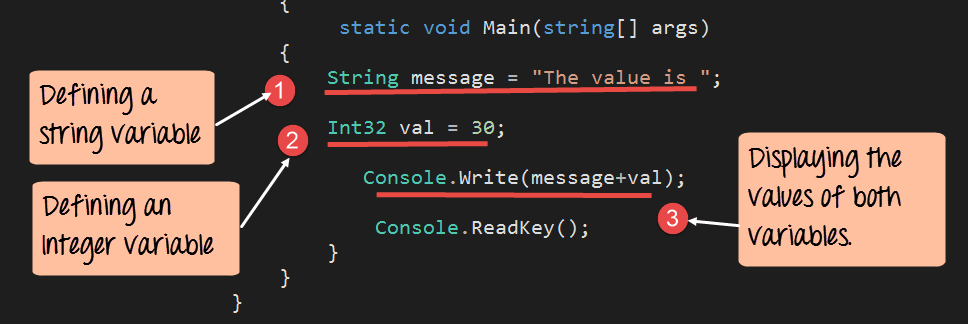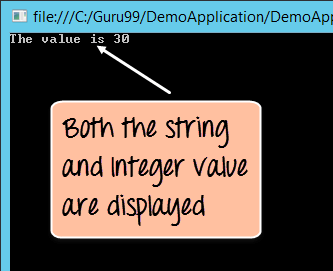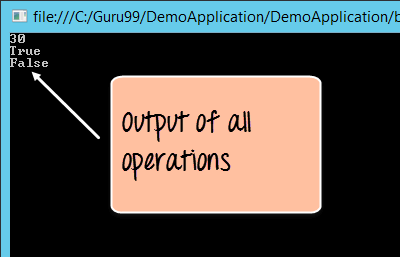# C# Variables operator

## C# Variables

A variable is a name given to a storage area that is used to store values of various data types. Each variable in C# needs to have a specific type, which determines the size and layout of the variable’s memory.

For example, a variable can be of the type String, which means that it will be used to store a string value. Based on the data type, specific operations can be carried out on the variable.

For instance, if we had an Integer variable, then operations such as addition and subtraction can be carried out on the variable. One can declare multiple variables in a program.

Let’s look at a quick example of the declaration of multiple variables of different data types.

In our example, we will define two variables, one of the type ‘string’ and the other of the type ‘Integer’. We will then display the values of these variables to the console. For each example, we will modify just the main function in our Program.cs file.```using System;
using System.Collections.Generic;
using System.Linq;
using System.Text;
namespace DemoApplication
{
class Program
{
static void Main(string[] args)
{
String message="The value is ";
Int32 val=30;

Console.Write(message+val);
}
}
}```

#### Code Explanation

1. A variable of the data type String is declared. The name of the variable is ‘message’. The value of the variable is “The value is “.
2. A variable of the data type Integer (Int32) is declared. The name of the variable is ‘val’. The value of the variable is 30.
3. Finally the Console.write statement is used to output both the value of the String and Integer variable.

If the above code is entered properly and the program is executed successfully, the following output will be displayed.

#### OutputFrom the output, you can see that the values of both the string and integer variable are displayed to the console.

Operators are used to performing operations on values of various data types. For example, to perform the addition of 2 numbers, the + operator is used.

Let’s see the table of operators available for the various data types

## C# Operators

### Arithmetic Operators

These are operators used for performing mathematic operations on numbers. Below is the list of operators available in C#.

 Operator Description + Adds two operands – Subtracts the second operand from the first * Multiplies both operands / Divides the numerator by de-numerator % Modulus Operator and a remainder of after an integer division ++ Increment operator increases integer value by one — Decrement operator decreases integer value by one

### Relational Operators

These are operators used for performing Relational operations on numbers. Below is the list of relational operators available in C#.

 Operator Description == Checks if the values of two operands are equal or not, if yes then condition becomes true. != Checks if the values of two operands are equal or not, if values are not equal then condition becomes true. > Checks if the value of left operand is greater than the value of right operand, if yes then condition becomes true. < Checks if the value of left operand is less than the value of right operand, if yes then condition becomes true. >= Checks if the value of left operand is greater than or equal to the value of right operand, if yes then condition becomes true. <= Checks if the value of left operand is less than or equal to the value of right operand, if yes then condition becomes true.

### Logical Operators

These are operators used for performing Logical operations on values. Below is the list of operators available in C#.

 Operator Description && This is the Logical AND operator. If both the operands are true, then condition becomes true. || This is the Logical OR operator. If any of the operands are true, then condition becomes true. ! This is the Logical NOT operator.

Let’s look at a quick example of how the operators can be used in .Net.

In our example, we will define 2 Integer variables and one Boolean variable. We will then perform the following operations```using System;
using System.Collections.Generic;
using System.Linq;
using System.Text;
namespace DemoApplication
{
class Program
{
static void Main(string[] args)
{
Int32 val1 = 10,val2 = 20;
bool status = true;

Console.WriteLine(val1 + val2);
Console.WriteLine(val1 < val2);
Console.WriteLine(!(status));
}
}
}```

#### Code Explanation

1. Two Integer variables are defined, one being val1 and the other being val2. These will be used to showcase relational and arithmetic operations. A Boolean variable is defined to showcase logical operations.
2. An example of the arithmetic operation is shown wherein the addition operator is carried out on val1 and val2. The result is written to the console.
3. An example of the relational operation is shown wherein the less than operator is carried out on val1 and val2. The result is written to the console.
4. An example of the logical operation is shown, wherein the logical operator (!) is applied to the status variable. The logical NOT operator reverses the current value of any Boolean value. So if a Boolean value is ‘true’, the logical NOT will return the value ‘false’ and vice versa. In our case since the value of the status variable is ‘true’, the result will show ‘false’. The result is written to the console.

If the above code is entered properly and the program is executed successfully, the output will be displayed.

#### Output